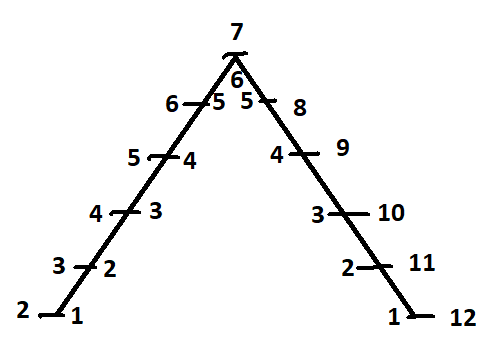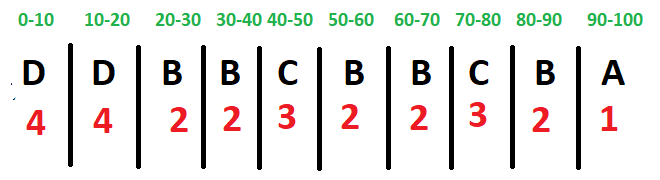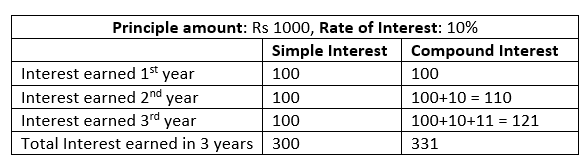# Quant Shortcut Tricks and Tips for CAT: Shortcut TechniquesThese quant shortcut tips and tricks for CAT will help you answer your questions in 10-15 seconds. We have tried to cover all the important topics of quant. So, do make your own notes.

## Quant Shortcut Tricks and Tips for CAT

We will cover the following quant shortcut tips and tricks for CAT:

• Tricks to solve Dice questions
• Tricks to find no of Prime numbers between 0-100

### Tricks to solve Dice questions in CAT exam

This trick is also called Triangle Trick. This trick is so simple that you can answer by simply looking at the question.

If you throw a dice twice, the total number of possible outcomes are 6 x 6 = 36. Similarly, if you throw two dice once, then again total number of possible outcomes are 6 x 6 = 36. So, throwing a dice twice or throwing two dice once is one and same thing. The number of cases are exactly same in both the cases.

The conventional method of answering the questions were to count all the possible outcome like (1,1), (1,2) and soon. But in this trick, you make a triangle like show below

The base of triangle is the minimum sum of outcomes of two dices i.e 2 and 12. The number on inner side of triangle represents the maximum number of cases of sum of outcomes of two dices.Lets’ take an example, if you throw two dices, what is the probability that sum of outcomes of two dices is 5. It is clear from above triangle that there are 4 cases for sum as 5. Therefore, probability comes out to be 4/36 i.e. 1/9.

Similarly, you can answer any question related to dice.

### Tricks to find no of Prime numbers between 0-100

The prime number is that number which has only two factors i.e. one factors is number 1 and other factor is the number itself. Also remember the lowest prime number is 2. The number one (1) is neither a prime number nor a composite number.

In exam, you will be asked following types of questions:

• What are the number of prime numbers between 0-100?
• What are the number of prime numbers between 50-70?

You can easily remember each prime number upto 20 or 30, but after that it is very difficult to remember which number is prime number.

The trick is to remember the code: D D B B C B B C B A and write it as per table shown below:As per table above, there are:

• 4 prime numbers between 0 to 10
• 4 prime numbers between 10 to 20
• 2 prime numbers between 20 to 30
• 2 prime numbers between 30 to 40
• 3 prime numbers between 40 to 50
• 2 prime numbers between 50 to 60
• 2 prime numbers between 60 to 70
• 3 prime numbers between 70 to 80
• 2 prime numbers between 80 to 90
• 1 prime numbers between 90 to 100

So, there are 25 prime numbers between 0-100.

Sample Question: How many prime numbers are there between 0 and 50?

Answer: Look at the table above and sum up the number upto 50. i.e. 4+4+2+2+3 = 15

Similarly, you can answer any questions related to number of prime number between 0 to 100.

### Tricks to solve Simple Interest and Compound Interest questions in CAT exam

This trick will help you solve any questions of simple interest and compound interest without using any formula.

In simple words, compound interest means interest on interest. Lets’ illustrate it with the help of an example. Assume you have Rs 1000 to invest for 3 years at rate of interest of 10%. Lets’ see the difference between simple interest and compound interest as shown below:In these type of questions, you just need to calculate the net interest rate.

Trick to calculate simple interest

From above table we can conclude that at simple interest:

• Interest earned after one year is Rs 100, which is 10% of principal amount
• Interest earned after two year is Rs 100 + Rs 100 = Rs 200, which is 20% of principal amount
• Interest earned after three year is Rs 100 + Rs 100 + Rs 100 = Rs 300, which is 30% of principal amount

We can easily identify that each year 10% interest is getting added. So, you just need to multiple rate of interest with number of years to get the total interest rate.

Sample Question: What is interest earned on sum of Rs 5000 lent at simple interest of 12% for 5 years?

Answer: In 5 years, total interest rate will become 12% x 5 = 60%, so our answer will be 60% of Rs 5000; which comes out to be Rs 3000.

Trick to calculate compound interest

In compound interest question also, the shortcut is to calculate the net interest rate. For example, to calculate compound interest on sum of Rs 1000 at simple interest of 10% per annum for 2 years, the trick to calculate compound interest is 10 + 10 + (10 x 10)/100 = 21. So your net  interest rate comes out to be 21%. 21% of Rs 1000 is Rs 210. So, your answer is  Rs 210.

Check out CAT Course 2022